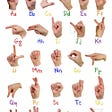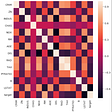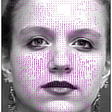# K Nearest Neighbor Algorithm: Explained from Scratch.

• KNN identifies the K number of neighbors.
• It calculayes the nearest neighbors by calculating the distance. The distance between two points in space is calculated by any of the distances such as Euclidean, Minkowski, Manhattan(putting q=1,2 in Minkiwoski gives Manhattan & Euclidian respectively).
• Now it does a probability test after calculating the number of neighbors.
• In our case there are 2 blue and 1 orange. P(B) =2/3 >P(O)=1/3.
• Hence the target is identified as Blue.
• KNN don’t have any training process basically or because it directly does the calculations on test data by finding the Euclidean Distance for the nearest K points.
• KNN can’t be used on big datasets because of the above point. If the data is very big then KNN will take a lot of time calculating the nearest neighbor of every point which is not at all practical.
• When the clusters overlap KNN collapse because KNN works only on two dimensions same is with Naive Bayes also. SVM overcomes this problem.

--

--

--

## More from Akshar Rastogi

Love podcasts or audiobooks? Learn on the go with our new app.

## The Role of The t-Student Distribution## Portfolio Diversification with Emerging Market Bonds## Hard data alone is just that: data## Competing in the Digital Age — Insurance Spotlight## Introducing Copula in Monte Carlo Simulation## MC 471 Assignment One## A Guide to become a Data Scientist/AI Expert## Data Analytics Advantages and Challenges## ML.NET and the magic of Model Builder using the ASL alphabet## Sabudh Foundation Internship 1st Month## Automatic Facial Expression Recognition Using Data Mining Techniques## Explain Like I’m Five (Part 2) : Support Vector Machine